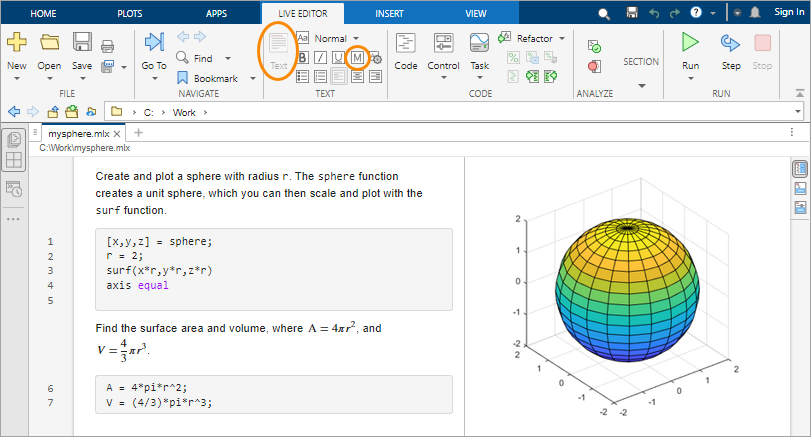## 编程和脚本

### 脚本

`edit mysphere`

```[x,y,z] = sphere; r = 2; surf(x*r,y*r,z*r) axis equal ```

```A = 4*pi*r^2; V = (4/3)*pi*r^3; ```

```% Create and plot a sphere with radius r. [x,y,z] = sphere; % Create a unit sphere. r = 2; surf(x*r,y*r,z*r) % Adjust each dimension and plot. axis equal % Use the same scale for each axis. % Find the surface area and volume. A = 4*pi*r^2; V = (4/3)*pi*r^3; ```

`mysphere`

### 实时脚本

• 将注释行转换为文本。选择以百分比符号开头的每一行，然后选择。删除百分比符号。

• 重写文本以替换代码行末尾的注释。要将等宽字体应用于文本中的函数名，请选择。要添加方程，请在选项卡上选择`edit newfile.mlx`

### 循环及条件语句

```N = 100; f(1) = 1; f(2) = 1; for n = 3:N f(n) = f(n-1) + f(n-2); end f(1:10) ```

```ans = 1 1 2 3 5 8 13 21 34 55 ```

```num = randi(100) if num < 34 sz = 'low' elseif num < 67 sz = 'medium' else sz = 'high' end ```

### 脚本位置

MATLAB 在特定位置中查找脚本及其他文件。要运行脚本，该文件必须位于当前文件夹或搜索路径中的某个文件夹内。